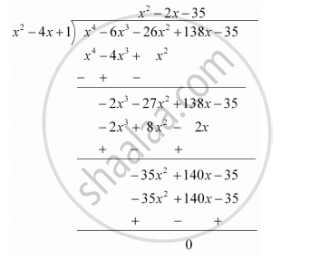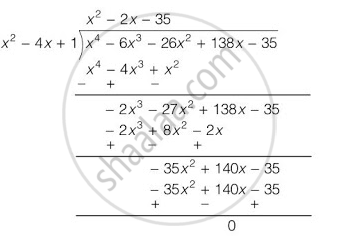# If two zeroes of the polynomial x^4 – 6x^3 – 26x^2 + 138x – 35 are 2 ±  √3, find other zeroes - Mathematics

If two zeroes of the polynomial x4 – 6x3 – 26x2 + 138x – 35 are 2 ± sqrt3 , find other zeroes

#### Solution 1

Given that 2 + sqrt3 and 2 - sqrt3 are zeroes of the given polynomial

Therefore, (x - 2-sqrt3)(x-2+sqrt3) =  x2 + 4 - 4x - 3

= x2 - 4x + 1 is a factor of the given polynomial

For finding the remaining zeroes of the given polynomial, we will find the quotient by dividing by x4 - 6x3 - 26x2 + 138x - 35 by x2 - 4x + 1Clearly x4 - 6x3 - 26x2 + 138x - 35 = (x2 - 4x +1)(x2 -2x -35)

It can be observed that (x2 - 2x - 35) is also a factor of the given polynomial.

And (x2 - 2x - 35) = (x -7)(x + 5)

Therefore, the value of the polynomial is also zero when x - 7 = 0 or x + 5 = 0

or x = 7 or -5

Hence, 7 and - 5 are also zeroes of this polynomial

#### Solution 2

2+√3 and 2-√3 are two zeroes of the polynomial p(x) = x4 – 6x3 – 26x2 + 138x – 35.

Let x = 2±√3

So, x-2 = ±√3

On squaring, we get x2 - 4x + 4 = 3,

Now, dividing p(x) by x2 - 4x + 1∴ p(x) = x4 - 6x3 - 26x2 + 138x - 35

= (x2 - 4x + 1) (x2 - 2x - 35)

= (x2 - 4x + 1) (x2 - 7x + 5x - 35)

= (x2 - 4x + 1) [x(x - 7) + 5 (x - 7)]

= (x2 - 4x + 1) (x + 5) (x - 7)

∴ (x + 5) and (x - 7) are other factors of p(x).

∴ - 5 and 7 are other zeroes of the given polynomial.
Concept: Relationship Between Zeroes and Coefficients of a Polynomial
Is there an error in this question or solution?
Chapter 2: Polynomials - Exercise 2.4 [Page 37]

#### APPEARS IN

NCERT Class 10 Maths
Chapter 2 Polynomials
Exercise 2.4 | Q 4 | Page 37

Share